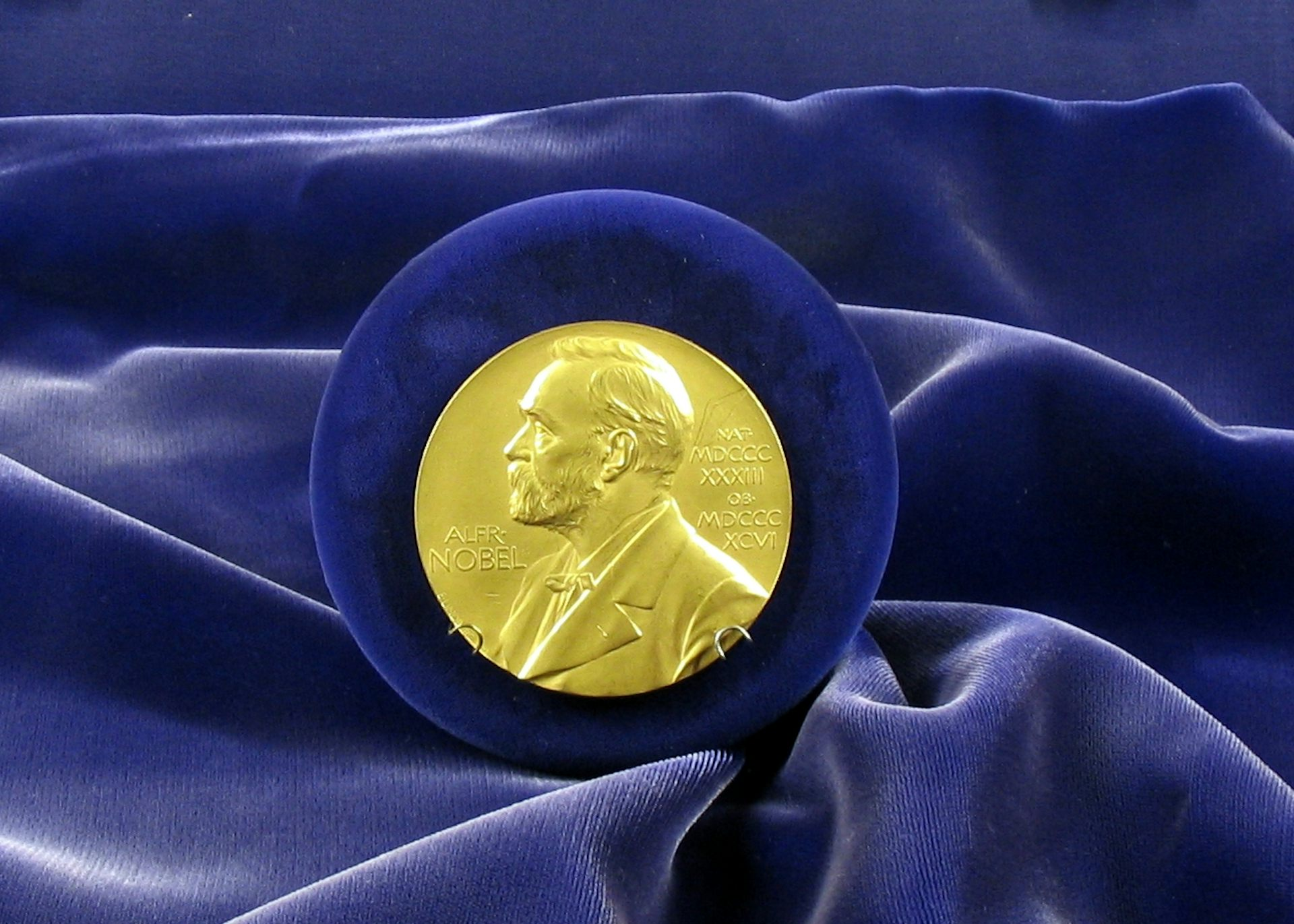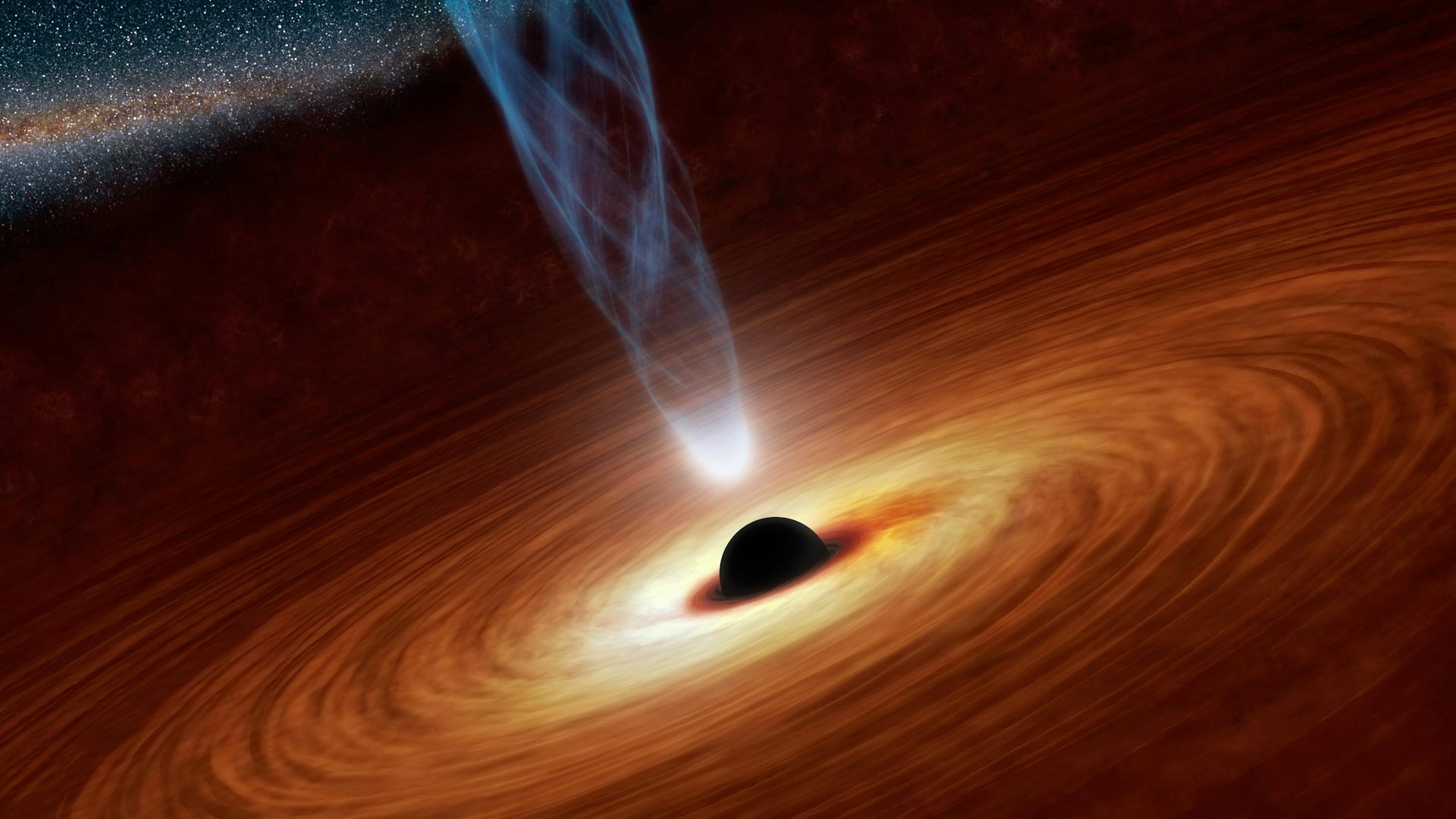# The Nobel Prize for Physics goes to topology – and mathematicians applaudMath doesn’t get its own Nobel but is the foundation for much Prize-winning research. Tim Ereneta, CC BY-NC

David ThoulessDuncan Haldane, and Michael Kosterlitz received the 2016 Nobel Prize for Physics for their work on exotic states of matter. They were inspired by the observation that some materials have unusual electrical properties – and their investigations led them to topology. That’s the branch of mathematics concerned with the properties of geometric objects that don’t change when bent or stretched (though torn would be a different story). As there is no Nobel Prize for mathematics, the topology community is understandably excited by this recognition of the utility of our discipline.

The old saw is that a topologist is a mathematician who cannot tell the difference between a doughnut and a coffee cup. (This joke is getting tiresome, but we stick with it anyway.) Both objects have just one hole and it’s easy to see how to deform one to the other.

Topology aims to classify these spaces via indirect means. Since it’s often rather difficult to demonstrate how to deform a particular space to make it look like another, topologists develop mathematical machinery that takes spaces as an input and produces an algebraic object. This output might just be a number or it could be more complicated, but the machine should take spaces that are “the same” and spit out the same result. This allows us to distinguish spaces – two inputs are different if the corresponding outputs are different.

For example, it may seem obvious that a doughnut and a sphere are distinct objects, but just because you cannot see how to deform one to the other it doesn’t follow that it’s impossible. Topology comes to the rescue, however. One of many ways to show that a sphere and a doughnut aren’t the same is to compute their fundamental groups. This is an algebraic object built from considering loops in the space.

A useful way to visualize loops is to imagine a rubber band lying on the surface of an object. First , consider the sphere. Any loop on the sphere contains a disc inside it, and now you can imagine shrinking that loop down to a single point by pulling it through the disc. So there aren’t any interesting loops on the sphere – they are all deformable to a single point.

That’s not true for the doughnut, however. In fact ,there are lots of interesting loops on its surface (we are dealing with a hollow doughnut; there’s nothing but air inside). One such loop is obtained by drawing a circle around a vertical cross section (the blue loop in the figure below). Another arises from a horizontal cross-section (the red loop). It’s impossible to contract these loops down to a the same single point, so the fundamental groups of the sphere and doughnut aren’t the same and thus, they are different objects.

## The topology of materials

Topology works in all dimensions, but physics is mostly concerned with our three-dimensional universe (well, that’s not always true – just ask string theorists). When studying electrical properties of materials, we are definitely dealing with three dimensions. Even a thin wire has length, width and height. For a fixed electrical conductor, say a copper wire, it’s usually possible to determine the relationship between the voltage placed on the wire and the current that flows. Sometimes, however, materials experience an electrical phase transition (superconductivity, for example, which is obtained by lowering the temperature of the material) and the usual equations governing voltage and current break down.

Thouless, Haldane and Kosterlitz discovered that mathematically these transitionscorrespond to an abrupt change in the topological type of the material. Certain thin films can be considered as being two-dimensional – imagine a surface that’s only one atom thick – and electrical current often flows in channels on the surface with low resistance. It turns out that there are points where the electrons flow around in a circular motion, sometimes clockwise and sometimes counterclockwise, and the number of such points can change as the material undergoes a phase transition.

Mathematicians immediately recognize this type of space from a first course in algebraic topology – it’s a plane with a few points removed and its fundamental group is very easy to compute. It turns out that the number of these types of points completely determines the topological type of the space.How can you scientifically describe a black hole without math? NASA/JPL-Caltech, CC BY

## Topology elsewhere in physics

Einstein’s general theory of relativity posits that space-time is curved by gravity. The equations also imply the existence of black holes, which in mathematical terms correspond to singularities, points in a space where all hell breaks loose (so to speak). A typical example familiar to calculus students is a point on the graph of a function where the derivative fails to exist. Much more complicated examples are possible and the space around such points can have interesting topology. Around ordinary points, space looks like a three-dimensional ball, but around singularities ,space can be knotted in unusual ways. Of course, we can’t experience this ourselves, but we can model it mathematically.

Topology has provided a framework in physics in other ways, such as the development of topological quantum field theoriesString theory is a generalization of this idea in which particles are modeled by one-dimensional objects called strings. These theories, unlike Einstein’s four-dimensional spacetime, require extra dimensions to be consistent – either 10, 11 or 26 depending on which model you prefer. Why don’t we observe these dimensions? The prevailing interpretation is that they are “small” and curl up on themselves so that we don’t notice. These extra dimensions form a type of space familiar to algebraic geometers called a Calabi-Yau manifold.

So it seems that a great deal of theoretical physics is based in sophisticated mathematics. Using ideas from topology, algebraic geometry and abstract algebra, not to mention differential equations and probability, physicists attempt to make sense of our universe. While math may not have its own Nobel Prize, many of the significant advances in other disciplines would not be possible without the development of sophisticated mathematics to provide the proper language for stating the results (Heisenberg’s uncertainty principle, for example).

This is all heady stuff. In the end, though, the discoveries made by Thouless, Haldane and Kosterlitz have led to practical devices currently in use in industry (for example, efficient hard drives in computers) and may lead to advances in quantum computing. Understanding how electrons move in materials is crucial to building better computers and instruments, and it’s exciting for us mathematicians to know that topology can help get us there.